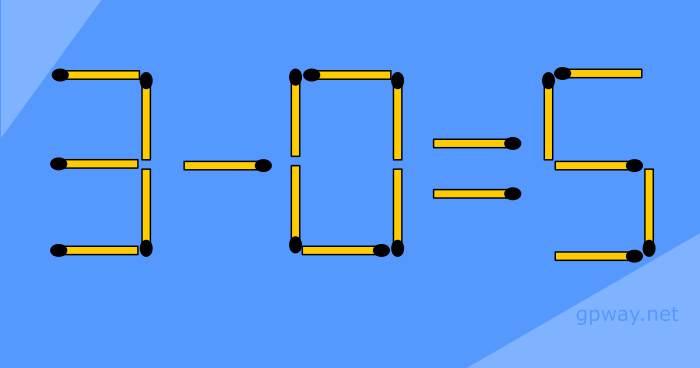# Matchstick Puzzles 1

Love puzzles then try some of these
Try these old matchstick puzzles or just enjoy solving or ask friends to solve withing limited times. Some puzzles are hard some are too easy. Solving these problems are still fun.

# Matchstick puzzles

This post contains the first series of matchstick puzzle which is very exciting for many people. They enjoy solving and asking to solve the same among friends.

### Move one match to make a squareAs shown in the image above, four matchsticks are placed in a way it creates mathematicle sign plus. Your task is to make a square by moving only one matchstick.
Square has four equal straight sides and four right angles. See the answer here

### Move two matchsticks to make this equation trueMatchsticks here form mathematicle equation and your task is to correct it. The equation given here is false you have to make it true by moving two matchsticks only. See the answer here

### Add two matchsticks to make this equation trueThese matchsticks shows mathematicle equation your task is to correct it. You are allowed to add more two matchsticks to make this equation true. See the answer here